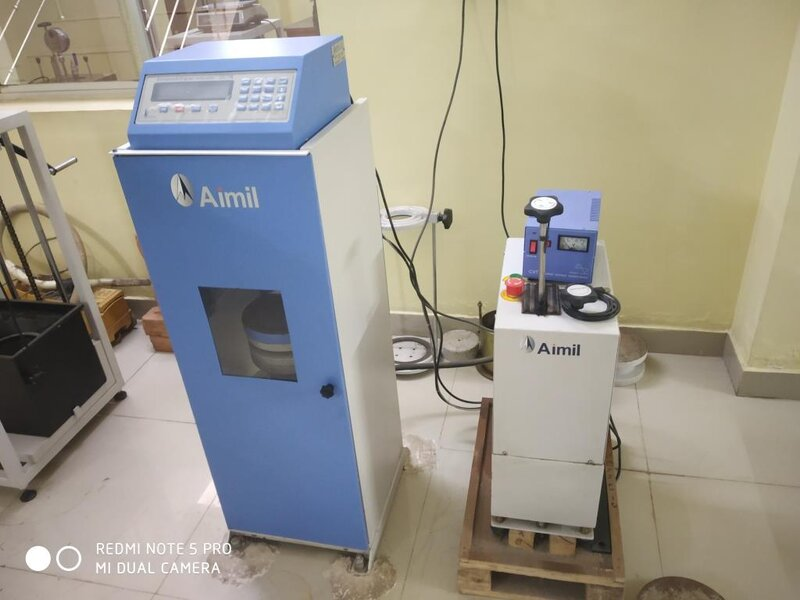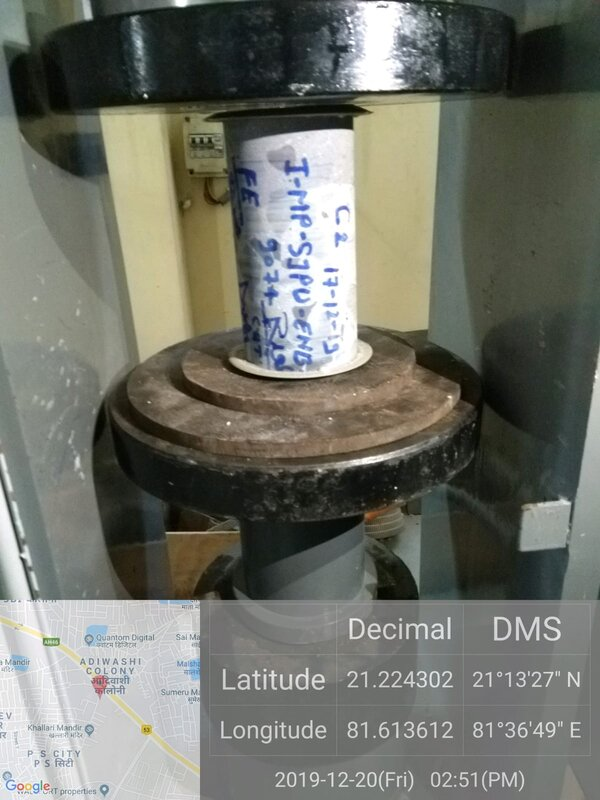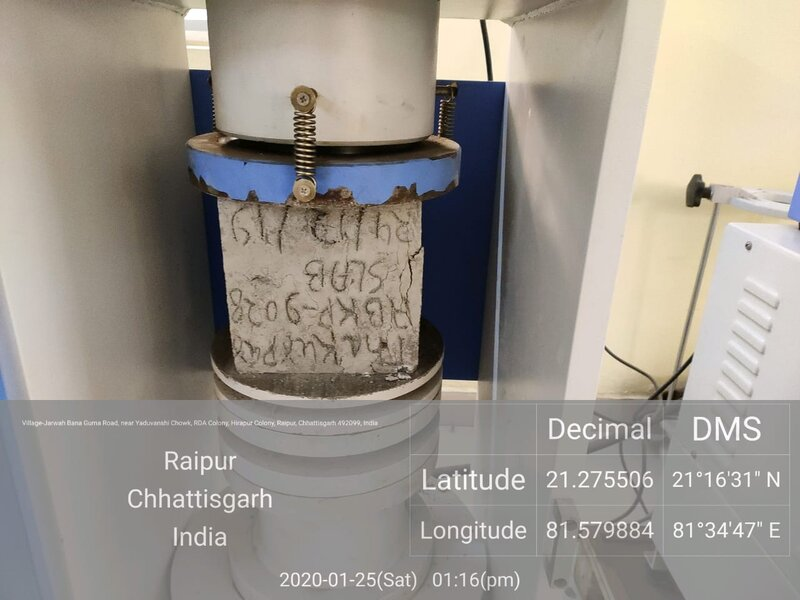# Core and Cube Test Procedure loading rate calculations as per IS 516

#### saroja kumar swain

##### Newbie
IS 516-1959 page no 20 section 9.8Procedure- The Load shall be applied continuously and without shock at a rate of 140 Kg/Sq cm/min.

In machine load applied KN/Sq mm /sec.
1st convert kg/sq cm/min to KN/ Sq mm/sec.
1 kg=0.0098 KN, 1 Sq cm= 100 Sq mm, 1 Min = 60 sec
so 140 Kg/Sq cm/min= (140*0.0098)/100/60=(140*0.0098)/(100*60)=0.00022867=0.0002287 KN/ Sq mm/secCORE=69 mm
Surface Area of Core= π * r 2= 3.14 * 34.5 * 34.5 = 3737.385 Sq mm
Rate of loading per Second in KN for Core = 0.0002287 Kn/Sq mm/sec * 3737 Sq mm = 0.85473 Kn/secCUBE =100*100 mm
Area of cube=100 * 100=1000 Sq mm
Rate of loading per second in KN for cube test size 100 * 100= (0.0002287 Kn/Sq mm/sec) * 1000 Sq mm = 2.287 Kn/sec

CUBE=150*150 mm

Area of cube=150 * 150 = 22500 Sq mm
Rate of loading per second in KN for cube test size 150 * 150= (0.0002287 Kn/Sq mm/sec) * 22500 Sq mm = 5.14 Kn/sec
Rate of loading per Minute =5.14 Kn/Sec * 60 = 308.7 KN/Minute

#### saroja kumar swain

##### Newbie
Note in core procedure in punching mistake
CORE=69 mm
Surface Area of Core= π *r2= 3.14*34.5*34.5=3737.385 Sq mm
Rate of loading per Second in KN for Core =0.0002287 Kn/Sq mm/sec *3737 Sq mm = 0.85473 Kn/sec

#### T Kolundu

##### Newbie
Since, the machine should have the capability of "servo control mechanism"; that is slightly expensive. If it is not, then you may have to control manually to the nearest.
eg. CC cubes - 5.1 kN /s

#### Shivaraj okali

##### Newbie
Note in core procedure in punching mistake
CORE=69 mm
Surface Area of Core= π *r2= 3.14*34.5*34.5=3737.385 Sq mm
Rate of loading per Second in KN for Core =0.0002287 Kn/Sq mm/sec *3737 Sq mm = 0.85473 Kn/sec
Sir how to convert kn/mm2 to kg/cm2

Replies
0
Views
139
Replies
0
Views
1,057
Replies
0
Views
437
Replies
0
Views
453
Replies
4
Views
883